# Train

The train is running at speeds of 96 km/h. From the beginning of braking to full stop train run for 3.3 minutes. If the train slows the braking equally, calculate the distance from the location where you need to start to brake.

s =  2640 m

### Step-by-step explanation: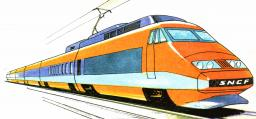Did you find an error or inaccuracy? Feel free to write us. Thank you!Tips to related online calculators
Do you want to convert length units?
Do you want to convert velocity (speed) units?

#### You need to know the following knowledge to solve this word math problem:

We encourage you to watch this tutorial video on this math problem:

## Related math problems and questions:

• An accelerationThe car goes on a straight road at a speed of 72 km/h. At some point, the driver starts to brake and stops the car in 5 seconds. Find: (a) the acceleration during braking (b) the distance traveled during braking.
• Train speed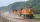The train speed is decreased during 50 sec from 72 km/h to 36 km/h. Assuming that the train movement is equally slowing, find the the acceleration and the distance that it travels at.
• Reconstruction of the corridorCalculate how many minutes will be reduced to travel 187 km long railway corridor, where the maximum speed increases from 120 km/h to 160 km/h. Calculate how many minutes will shorten travel time, if we consider that the train must stop at 6 stations, eac
• Braking distance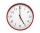The car travels at an average speed of 12 km/h and detects an obstacle 10 m in front of it. At 1 m in front of the obstacle it already runs 2 km/h. What is the braking distance? What is the required deceleration for stop in: A) 1m B) 1s?
• Train 2The train slowed down from 90 km/h to 72 km/h in 5 seconds. How long track travel?
• BrakesThe braking efficiency of a passenger car is required to stop at 12.5 m at an initial speed of 40 km/h. What is the acceleration braking by brakes?The railroad runs parallel to the railway. The train travels at 36 km/h on track. In the opposite direction, the motorcycle rides at 90 km/h. The train passes in 3 seconds. How long is a train?
• Two trains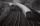There are two trains running the same distance. 1st train will travel it in 7 hours 21minutes. 2nd the train will travel 5 hours 57minutes and 34 seconds and it is 14 km/h faster than the first train. What are speeds of trains and how long is this railway
• Train delay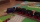Due to a breakdown, the train lost 16 minutes of standing on the track behind Brno. He "eliminated" this delay so that after the start, the 80 km long section went at a speed 10 km/h higher than originally planned. What speed was it and what was it suppos
• Cars motion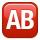From point A car start at the speed of 90 km/h. Against it departs from the point B at the same time another car at 60 km/h. For how long and where they met if the distance between A and B is 180 km?
• DriverThe car driver drives at a speed of 100 km/h, saw the obstacle, and began to brake with a slowing of 5 m/s². What is the length of the pathway to stopping the car when the driver has registered the obstacle with a delay of 0.7 s?
• Bus vs. trainThe bus started from point A 10 minutes before the train started from the same place. The bus went an average speed of 49 km/h, train 77 km/h. To point B train and bus arrived simultaneously. Calculate the time of the train journey, if train and bus trave
• Bus stop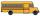Determine at what time the bus leaves from the bus stop outside the village, if you know that when you leave home at 8:00 and go at speed 3 km/h, you come to a stop 9 minutes after the departure of the bus, and when you go speed at 4 km/h, you come to the
• Two trainsThrough the bridge, long l = 240m, the train passes through the constant speed at time t1 = 21s. A train running along the traffic lights at the edge of the bridge passes the same speed at t2 = 9s. a) What speed v did the train go? b) How long did it take
• Traffic collisionWhen investigating a traffic accident, it was found that the driver stopped the vehicle immediately after the accident by constant braking on a 150 m track in 15 seconds. Do you approve that the driver exceeded the permitted speed (50 km/h) in the village
• G forcesCalculate deceleration of car (as multiple of gravitational acceleration g = 9.81 m/s2) occurs when a vehicle in a frontal collision slows down uniformly from a speed 111 km/h to 0 km/h in 1.2 meters trajectory.
• Runner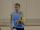Peter ran a speed of 12.4 km / h. After 45 minutes running he had a hiatus. The track is 31 km long. How many kilometers he still to run if he still running at the same speed?